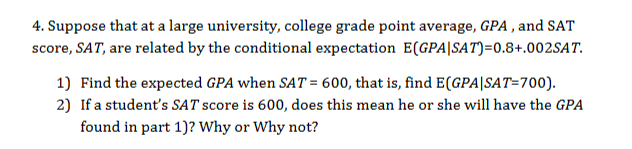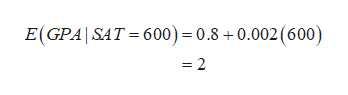4. Suppose that at a large university, college grade point average, GPA , and SATscore, SAT, are related by the conditional expectation E(GPA|SAT)=0.8+.002SAT1) Find the expected GPA when SAT = 600, that is, find E(GPA|SAT=700)2) If a student's SAT score is 600, does this mean he or she will have the GPAfound in part 1)? Why or Why not?

Question

Part 1 and 2help_outlineImage Transcriptionclose4. Suppose that at a large university, college grade point average, GPA , and SAT score, SAT, are related by the conditional expectation E(GPA|SAT)=0.8+.002SAT 1) Find the expected GPA when SAT = 600, that is, find E(GPA|SAT=700) 2) If a student's SAT score is 600, does this mean he or she will have the GPA found in part 1)? Why or Why not? fullscreen
Step 1

Note:

We consider E(GPA|SAT=700) as E(GPA|SAT=600) in part 1).

It is given that the conditional expectation is given as E(GPA|SAT) = 0.8 + 0.002 SAT.

Step 2

1) Expected GPA when SAT = 600:

The expected GPA when SAT = 600 is found t...help_outlineImage TranscriptioncloseE(GPA SAT 600) = 0.8+0.002 (600) =2 fullscreen

Want to see the full answer?

See Solution

Want to see this answer and more?

Our solutions are written by experts, many with advanced degrees, and available 24/7

See Solution
Tagged in

Other